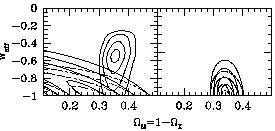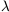4.5 Other sources of dark energy

Although a cosmological constant is an excellent fit to the current data, the observations can also be accommodated by any form of ``dark energy'' which does not cluster on small scales (so as to avoid being detected by measurements ofM) and redshifts away only very slowly as the universe expands [to account for the accelerated expansion, as per equation (32)]. This possibility has been extensively explored of late, and a number of candidates have been put forward.

One way to parameterize such a component X is by an effective equation of state, pX = wXX. (A large number of phenomenological models of this type have been investigated, starting with the early work in [206, 207]; see [208, 8] for many more references.) The relevant range for wX is between 0 (ordinary matter) and -1 (true cosmological constant); sources with wX > 0 redshift away more rapidly than ordinary matter (and therefore cause extra deceleration), while wX < -1 is unphysical by the criteria discussed in Section 2.1 (although see ). While not every source will obey an equation of state with wX = constant, it is often the case that a single effective wX characterizes the behavior for the redshift range over which the component can potentially be observed.Figure 10. Limits from supernovae and large-scale structure data onM and the equation-of-state parameter wX, in a flat universe dominated by matter and dark energy. Thin contours (on the left) represent limits from CMB and large-scale structure measurements, while thick contours are those from SNe observations; solid lines apply to models with constant wX, while dashed lines apply to models of dynamical scalar fields. The constraints are portrayed separately on the left, and combined on the right. From .

Current observations of supernovae, large-scale structure, gravitational lensing, and the CMB already provide interesting limits on wX [210, 211, 212, 213, 214, 49, 52, 215, 216, 217, 218, 219], and future data will be able to do much better [218, 220, 221, 222]. Figure (10) shows an example, in this case limits from supernovae and large-scale structure on wX andM in a universe which is assumed to be flat and dominated by X and ordinary matter. It is clear that the favored value for the equation-of-state parameter is near -1, that of a true cosmological constant, although other values are not completely ruled out.

The simplest physical model for an appropriate dark energy component is a single slowly-rolling scalar field, sometimes referred to as ``quintessence'' [223, 224, 225, 226, 227, 228, 229, 230, 231, 232, 233, 234]. In an expanding universe, a spatially homogeneous scalar with potential V() and minimal coupling to gravity obeys(62)

where H is the Hubble parameter, overdots indicate time derivatives, and primes indicate derivatives with respect to. This equation is similar to (45), with analogous solutions. The Hubble parameter acts as a friction term; for generic potentials, the field will be overdamped (and thus approximately constant) when H > sqrt[V''()], and underdamped (and thus free to roll) when H < sqrt[V''()]. The energy density is= (1/2)2 + V(), and the pressure is p= (1/2)2 - V(), implying an equation of state parameter(63)

which will generally vary with time. Thus, when the field is slowly-varying and2 << V(), we have w ~ -1, and the scalar field potential acts like a cosmological constant.

There are many reasons to consider dynamical dark energy as an alternative to a cosmological constant. First and foremost, it is a logical possibility which might be correct, and can be constrained by observation. Secondly, it is consistent with the hope that the ultimate vacuum energy might actually be zero, and that we simply haven't relaxed all the way to the vacuum as yet. But most interestingly, one might wonder whether replacing a constant parameterwith a dynamical field could allow us to relieve some of the burden of fine-tuning that inevitably accompanies the cosmological constant. To date, investigations have focused on scaling or tracker models of quintessence, in which the scalar field energy density can parallel that of matter or radiation, at least for part of its history [232, 235, 236, 237, 238, 239, 240]. (Of course, we do not want the dark energy density to redshift away as rapidly as that in matter during the current epoch, or the universe would not be accelerating.) Tracker models can be constructed in which the vacuum energy density at late times is robust, in the sense that it does not depend sensitively on the initial conditions for the field. However, the ultimate valuevac ~ (10-3 eV)4 still depends sensitively on the parameters in the potential. Indeed, it is hard to imagine how this could help but be the case; unlike the case of the axion solution to the strong-CP problem, we have no symmetry to appeal to that would enforce a small vacuum energy, much less a particular small nonzero number.

Quintessence models also introduce new naturalness problems in addition to those of a cosmological constant. These can be traced to the fact that, in order for the field to be slowly-rolling today, we require sqrt[V''(0)] ~ H0; but this expression is the effective mass of fluctuations in, so we have(64)

By particle-physics standards, this is an incredibly small number; masses of scalar fields tend to be large in the absence of a symmetry to protect them. Scalars of such a low mass give rise to long-range forces if they couple to ordinary matter; sincedoes couple to gravity, we expect at the very least to have non-renormalizable interactions suppressed by powers of the Planck scale. Such interactions are potentially observable, both via fifth-force experiments and searches for time-dependence of the constants of nature, and current limits imply that there must be suppression of the quintessence couplings by several orders of magnitude over what would be expected [241, 242, 243]. The only known way to obtain such a suppression is through the imposition of an approximate global symmetry (which would also help explain the low mass of the field), of the type characteristic of pseudo-Goldstone boson models of quintessence, which have been actively explored [230, 231, 244, 245, 246, 247]. (Cosmological pseudo-Goldstone bosons are potentially detectable through their tendency to rotate polarized radiation from galaxies and the CMB [241, 248]. See  for a discussion of further fine-tuning problems in the context of supersymmetric models.

Nevertheless, these naturalness arguments are by no means airtight, and it is worth considering specific particle-physics models for the quintessence field. In addition to the pseudo-Goldstone boson models just mentioned, these include models based on supersymmetric gauge theories [250, 251], supergravity [252, 253], small extra dimensions [254, 255], large extra dimensions [256, 257], and non-minimal couplings to the curvature scalar [258, 259, 260, 261, 262, 263, 264, 265, 266]. Finally, the possibility has been raised that the scalar field responsible for driving inflation may also serve as quintessence [267, 268, 269, 270], although this proposal has been criticized for producing unwanted relics and isocurvature fluctuations .

There are other models of dark energy besides those based on nearly-massless scalar fields. One scenario is ``solid'' dark matter, typically based on networks of tangled cosmic strings or domain walls [272, 273, 274, 275]. Strings give an effective equation-of-state parameter wstring = -1/3, and walls have wwall = -2/3, so walls are a better fit to the data at present. There is also the idea of dark matter particles whose masses increase as the universe expands, their energy thus redshifting away more slowly than that of ordinary matter [276, 277] (see also ). The cosmological consequences of this kind of scenario turn out to be difficult to analyze analytically, and work is still ongoing.